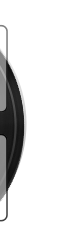1196.23万
VIP# 我的世界之史蒂夫冒险（第一辑）锋叔叔

【最全】我的世界 节目单指引

▲ 畅销超千万册，全球孩子都爱的冒险故事

▲ 真正打开孩子眼界，让想象力天马行空•53481826

官方喵现身啦😊想加入【我的世界】粉丝群的小朋友，请添加小助手微信【xmls0815】！进群不仅能遇到许多小伙伴，还有锋叔叔亲临互动哟～快来行动吧！

•子陌Zimo

我抽奖居然抽到了免费的！一听就发现真的太好听了！我现在每天都会收听这个！一听就停不下来了！大大的赞👍！！！！！！！！！

•小糊涂君

主播声音好听，故事情节引人入胜。

•我的老家就在这个屯儿

我的世界改编音频后居然还有这么棒的效果 非常意外的惊喜 大人孩子都可以听

•孟想孟梓仁•晓香3

我免费领取。 好好好好好好好好好好好好^_^^_^^_^^_^^_^^_^^_^^_^^_^^_^^_^^_^^_^^_^^_^^_^^_^^_^^_^^_^^_^^_^^_^^_^^_^^_^^_^^_^^_^^_^^_^^_^^_^^_^^_^^_^^_^^_^^_^^_^^_^

•魔皇HiM

锋叔叔，给你5星好评

•1376630kymf

很好😁太丰富#@好的grhr&&*就

•贾贝西

好好好好好好好好好好好好好好好好好好好好好好好好好好好好好好好好好好好好好好好好好好好好好好好好好好好好好好好好

•丨零丨零丨小号

666666666666666666666666666666666666666666666666666666666666